# Class 11 Maths NCERT Solutions for Chapter 16 Probability Miscellaneous Exercise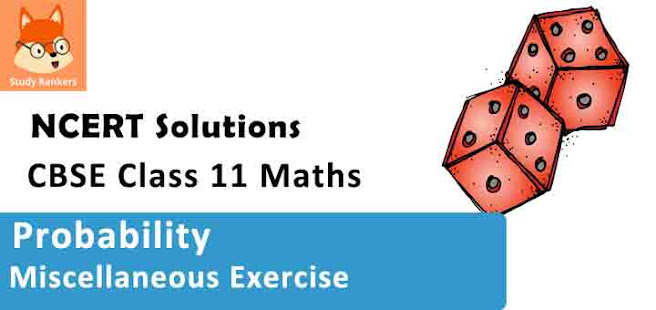### Statistics Miscellaneous Exercise Solutions

1.  A box contains 10 red marbles, 20 blue marbles and 30 green marbles. 5 marbles are drawn from the box, what is the probability that
(i) all will be blue?
(ii) atleast one will be green?

Solution

Total number of marbles = 10 + 20 + 30 = 60
Number of ways of drawing 5 marbles from 60 marbles = 60C5

(i) All the drawn marbles will be blue if we draw 5 marbles out of 20 blue marbles.
5 blue marbles can be drawn from 20 blue marbles in 20C5 ways.
∴ Probability that all marbles will be blue = 20C5/60C5

(ii) Number of ways in which the drawn marble is not green = (20 + 10)C5 = 30C5
∴ Probability that no marble is green = 30C5 / 60C5
∴ Probability that at least one marble is green = 1 – (30C5/60C5)

2. 4 cards are drawn from a well-shuffled deck of 52 cards. What is the probability of obtaining 3 diamonds and one spade?

Solution

Number of ways of drawing 4 cards from 52 cards = 52C4
In a deck of 52 cards, there are 13 diamonds and 13 spades.
∴ Number of ways of drawing 3 diamonds and one spade = 13C3 × 13C1
Thus, the probability of obtaining 3 diamonds and one spade =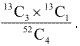3. A die has two faces each with number ‘1’, three faces each with number ‘2’ and one face with number ‘3’. If die is rolled once, determine
(i) P(2)
(ii) P(1 or 3)
(iii) P(not 3)

Solution

Total number of faces = 6

(i) Number faces with number ‘2’ = 3
∴ P(2) = 3/6 = 1/2

(ii) P (1 or 3) = P (not 2) = 1 − P (2) = 1 - 1/2 = 1/2

(iii) Number of faces with number ‘3’ = 1
∴ P(3) = 1/6
Thus, P(not 3) = 1 - P(3) = 1 - 1/6 = 5/6

4. In a certain lottery, 10,000 tickets are sold and ten equal prizes are awarded. What is the probability of not getting a prize if you buy (a) one ticket (b) two tickets (c) 10 tickets?

Solution

Total number of tickets sold = 10,000
Number prizes awarded = 10

(i) If we buy one ticket, then
P (getting a prize) = 10/10000 = 1/1000
∴ P (not getting a prize) = 1 – 1/1000 = 999/1000

(ii) If we buy two tickets, then
Number of tickets not awarded = 10,000 − 10 = 9990
P (not getting a prize) = 9990C2/10000C2

(iii) If we buy 10 tickets, then
P (not getting a prize) = 9990C10/10000C10.

5. Out of 100 students, two sections of 40 and 60 are formed. If you and your friend are among the 100 students, what is the probability that
(a) you both enter the same sections?
(b) you both enter the different sections?

Solution

My friend and I are among the 100 students.
Total number of ways of selecting 2 students out of 100 students = 100C2

(a) The two of us will enter the same section if both of us are among 40 students or among 60 students.
∴ Number of ways in which both of us enter the same section = 40C2 + 40C2
∴ Probability that both of us enter the same section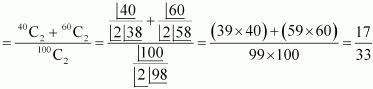(b) P(we enter different sections)
= 1 - P(we enter the same section)
= 1 - 17/33 = 16/33

6. Three letters are dictated to three persons and an envelope is addressed to each of them, the letters are inserted into the envelopes at random so that each envelope contains exactly one letter. Find the probability that at least one letter is in its proper envelope.

Solution

Let L1, L2, L3 be three letters and E1, E2, and E3 be their corresponding envelops respectively.
There are 6 ways of inserting 3 letters in 3 envelops. These are as follows: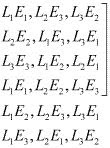There are  4 ways in which at least one letter is inserted in a proper envelope.
Thus, the required probability is 4/6 = 2/3.

7. A and B are two events such that P(A) = 0.54, P(B) = 0.69 and P(A ∩ B) = 0.35.
Find
(i) P(A ∩ B)
(ii) P(A′ ∩ B′)
(iii) P(A ∩ B′) (iv) P(B ∩ A′)

Solution

It is given that P(A) = 0.54, P(B) = 0.69, P(A ∩ B) = 0.35

(i) We know that P (A ∪ B) = P(A) + P(B) − P(A ∩ B)
∴P (A ∪ B) = 0.54 + 0.69 − 0.35 = 0.88

(ii) A′ ∩ B′ = (A ∪ B)′ [by De Morgan’s law]
∴P(A′ ∩ B′) = P(A ∪ B)′ = 1 − P(A ∪ B) = 1 − 0.88 = 0.12

(iii) P(A ∩ B′) = P(A) − P(A ∩ B)
= 0.54 − 0.35= 0.19
(iv) We know that n(B ∩ A') = n(B) - n(A ∩ B)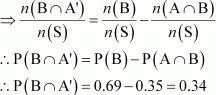8. From the employees of a company, 5 persons are selected to represent them in the managing committee of the company. Particulars of five persons are as follows:

 S. No. Name Sex Age in years 1. Harish M 30 2. Rohan M 33 3. Sheetal F 46 4. Alis F 28 5. Salim M 41
A person is selected at random from this group to act as a spokesperson. What is the probability that the spokesperson will be either male or over 35 years?

Solution

Let E be the event in which the spokesperson will be a male and F be the event in which the spokesperson will be over 35 years of age.
Accordingly, P(E) = 3/5 and P(F) = 2/5
Since there is only one male who is over 35 years of age,
P(E ∩ F) = 1/5
We know that P(E ∪ F) = P(E) + P(F) - P(E ∩ F)
∴ P(E ∪ F) = 3/5 + 2/5 - 1/5 = 4/5
Thus, the probability that the spokesperson will either be a male or over 35 years of age is 4/5.

9. If 4-digit numbers greater than 5,000 are randomly formed from the digits 0, 1, 3, 5, and 7, what is the probability of forming a number divisible by 5 when, (i) the digits are repeated? (ii) the repetition of digits is not allowed?

Solution

(i) When the digits are repeated
Since four-digit numbers greater than 5000 are formed, the leftmost digit is either 7 or 5.
The remaining 3 places can be filled by any of the digits 0, 1, 3, 5, or 7 as repetition of digits is allowed.
∴Total number of 4-digit numbers greater than 5000 = 2 × 5 × 5 × 5 − 1
= 250 − 1 = 249
[In this case, 5000 can not be counted; so 1 is subtracted]
A number is divisible by 5 if the digit at its units place is either 0 or 5.
∴Total number of 4-digit numbers greater than 5000 that are divisible by 5 = 2 × 5 × 5 × 2 − 1 = 100 − 1 = 99
Thus, the probability of forming a number divisible by 5 when the digits are repeated is = 99/249 = 33/83 .

(ii) When repetition of digits is not allowed
The thousands place can be filled with either of the two digits 5 or 7.
The remaining 3 places can be filled with any of the remaining 4 digits.
∴Total number of 4-digit numbers greater than 5000 = 2 × 4 × 3 × 2 = 48
When the digit at the thousands place is 5, the units place can be filled only with 0 and the tens and hundreds places can be filled with any two of the remaining 3 digits.
∴Here, number of 4-digit numbers starting with 5 and divisible by 5
= 3 × 2 = 6
When the digit at the thousands place is 7, the units place can be filled in two ways (0 or 5) and the tens and hundreds places can be filled with any two of the remaining 3 digits.
∴ Here, number of 4-digit numbers starting with 7 and divisible by 5
= 1 × 2 × 3 × 2 = 12
∴Total number of 4-digit numbers greater than 5000 that are divisible by 5 = 6 + 12 = 18
Thus, the probability of forming a number divisible by 5 when the repetition of digits is not allowed
is 18/48 = 3/8.

10. The number lock of a suitcase has 4 wheels, each labelled with ten digits i.e., from 0 to 9. The lock opens with a sequence of four digits with no repeats. What is the probability of a person getting the right sequence to open the suitcase?

Solution

The number lock has 4 wheels, each labelled with ten digits i.e., from 0 to 9.
Number of ways of selecting 4 different digits out of the 10 digits = 10C4 4
Now, each combination of 4 different digits can be arranged in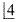ways.
Number of four digits with no repetitions =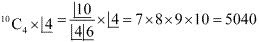There is only one number that can open the suitcase.
Thus, the required probability is  1/5040.